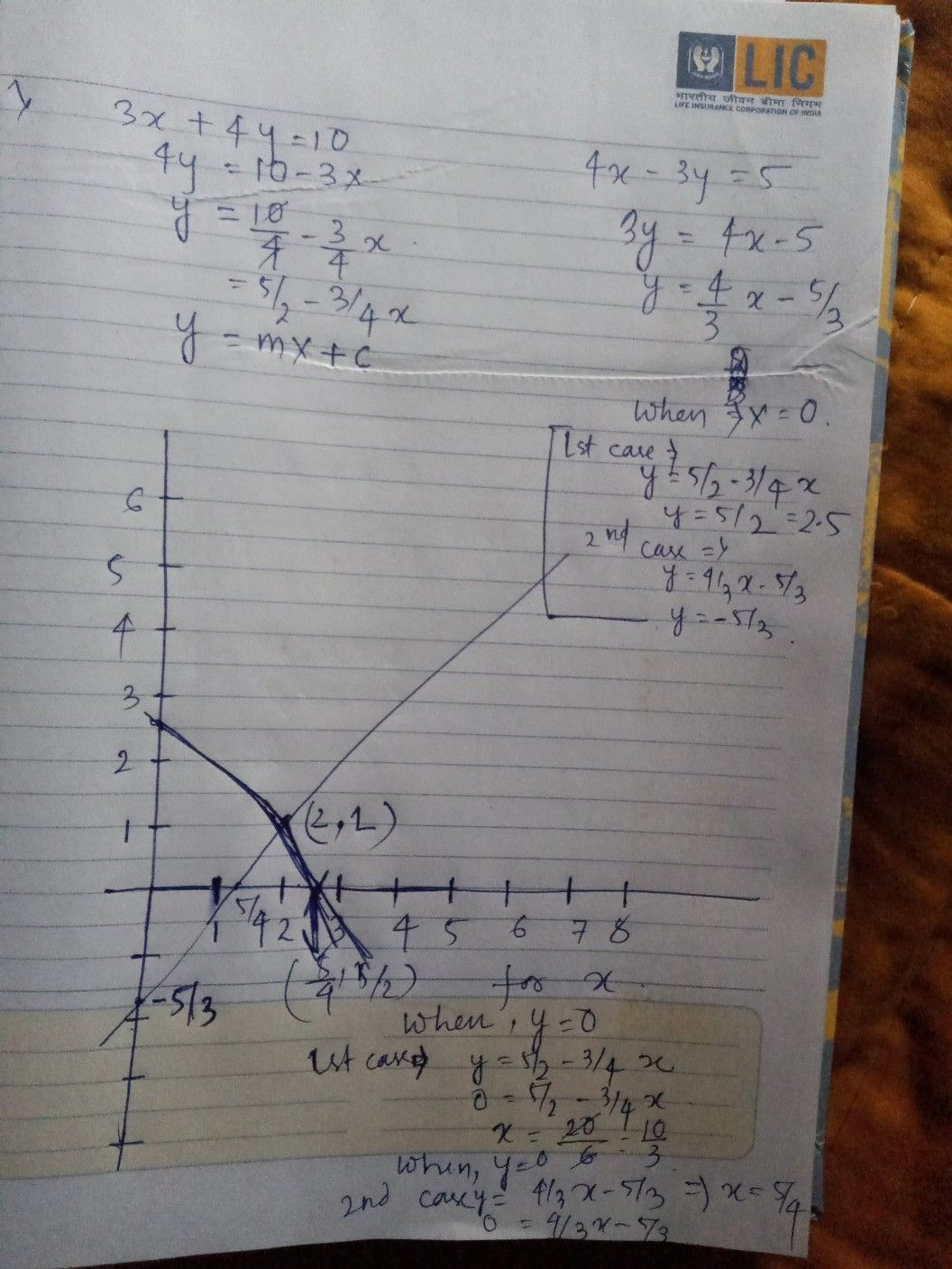Symbol
Problem$\square 2xQ$ (b) $\right)$ Solve the equations graphically $3x+4y=10$ and $4x-3y=5$ $.$
10th-13th grade
Other
Search count: 105
SolutionQanda teacher - NirupamaStudent
thank you mam